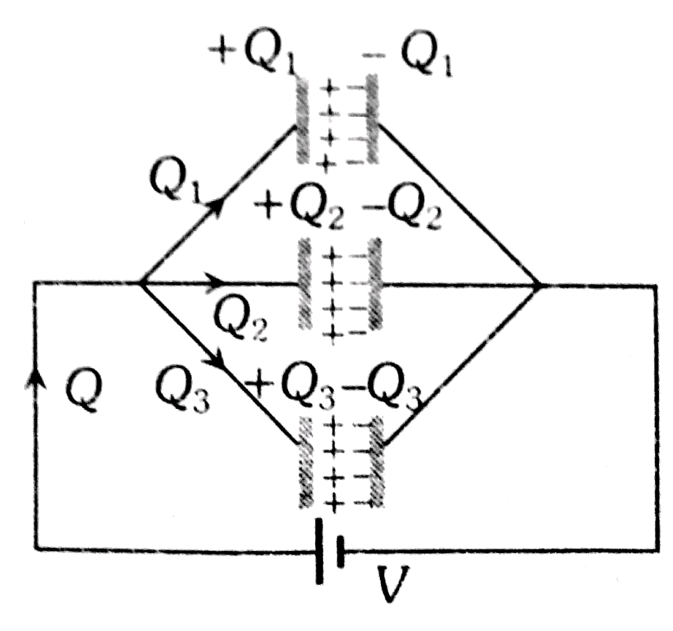Q

# Help me solve this In the circuit shown in the figure, each capacitor has a capacity of 3mF. The equivalent capacity between A and B is

In the circuit shown in the figure, each capacitor has a capacity of 3mF. The equivalent capacity between A and B is• Option 1)

• Option 2)

.

• Option 3)

• Option 4)

152 Views

As we have learned

Parallel Grouping -

- whereinHence equivalent capacitance

Option 1)

Option 2)

.

Option 3)

Option 4)

Exams
Articles
Questions